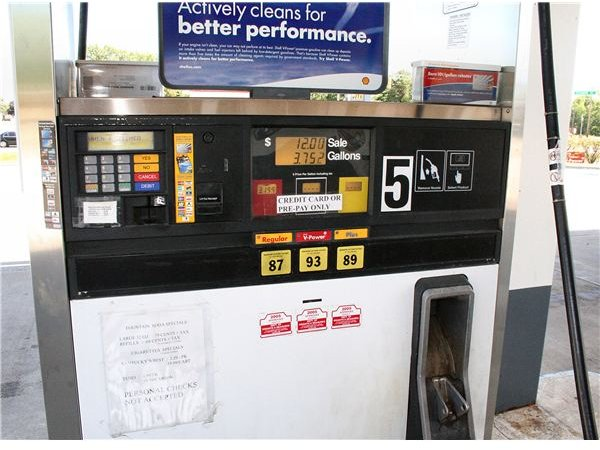# Figuring Out the Cost of Gas When Traveling: How To

Page content

## Introduction

When figuring out the cost of gas when traveling, it is important to understand the factors that contribute to this calculation. These factors include knowing the miles per gallon your vehicle your gets, the expected average cost for a gallon of gas, and the total distance you will travel during your trip. Since each of these numbers will vary depending on location and driving conditions, we will walk through some steps to ensure you are calculating the accurate amount of gas expenses for your trip.

The first step when estimating your fuel costs is to determine your vehicle’s average miles per gallon, also known as MPG. Since your vehicle gets better MPG when traveling at highway speeds versus city driving, you will need to figure out an average between these two numbers. There are many ways these numbers can be calculated, but a good website to find this information already calculated for you is Fuel Economy.gov.

If you prefer to figure out the fuel efficiency of your vehicle manually, a simple calculation method is to fill up your tank completely and make note of the odometer reading. When it’s time to fill your gas tank again, subtract the previous odometer reading from the current odometer reading. This number represents your miles per tank.

Next, you need to know the capacity of your gas tank measured in gallons. This number can usually be found in your owner’s manual or on the Internet, or you can call your dealership and they will furnish you with the capacity information. Once you have both of these numbers, you simply divide your miles per tank by the gas tank’s capacity to figure out your miles per gallon.Now that you have figured out your average MPG, you can begin figuring out the cost of gas when traveling. To determine the gas costs, you will need to know the approximate total miles you will be driving on your trip and the average price you expect to pay for a gallon of gas. To figure out the total fuel costs for your trip, use the following equation:

Distance ÷ MPG × Cost per Gallon = Total Fuel Cost

As another example, we will assume the total distance you will be traveling is 1000 miles, your vehicle averages 30 MPG, and the average cost per gallon of gas is \$3.00.

1000 ÷ 30 × \$3.00 = \$100

In the above example, the cost of gas for your trip would be \$100. To figure out the fuel cost for your trip, simply replace the numbers in the equation with accurate numbers for trip mileage, vehicle MPG, and expected price per gallon of fuel.

## Importance of Estimating the Cost of Gas

When planning a trip, figuring out the cost of gas when traveling will allow you to budget for more important things such as hotel rooms and recreational stops. For this reason, it makes sense to lean on the high side when making these calculations to avoid spending money you set aside for other elements of the trip. As a rule, always add ten percent to the total estimated fuel costs to prevent budget issues, especially since gas prices can raise unexpectedly during your trip. With proper planning and budgeting, you can easily figure out the cost of gas when traveling and keep the cost of your vacation in check.

“Calculating Your MPG” United States EPA: https://www.fueleconomy.gov/mpg/MPG.do?action=calcMP